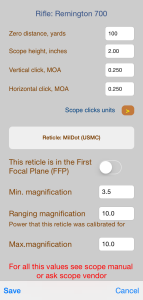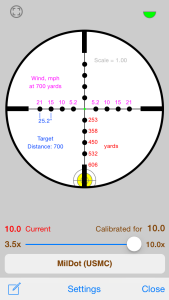﻿ Configuring and tuning iStrelok [iOS]

#Configuring and tuning iStrelok [iOS]

1. Enter Scope parameters2. Enter Cartridge name, bullet ballistic coefficient. (ballistic coefficients can be found here: Sierra, Hornady, Berger)

3. Measure bullet speed with chronograph and measure temperature of air and enter this values

4. Open Temperature sensitivity calculator, measure bullet speed at another temperature, tap "Calculate' button. Temperature sensitivity factor will be calculated. If you can not measure bullet speed, leave default value.

5. Zero your rifle and save you zeroing weather.

7. Now you are ready to shoot. Shoot to 700 yards distance

8. Do 'truing' by speed if needed.
Shoot at distance bigger than zero distance. Assume, this is 700 yards (Distance should be greater than the zero distance. The farther, the better). Calculated elevation for this distance is 81 clicks
Find out REAL elevation is needed to hit the target. Assume, this is 88 clicks.
On the trajectory validation screen input Distance as 700 yards and Elevation as 88 clicks.

Tap button "Calculate" and muzzle speed at which elevation will be equal 88 clicks will be calculated. It this case, it will be 2564 f/s
Tap button "Use this velocity" and this calculated muzzle speed will replace your current muzzle speed (2654->2564) and screen will be closed.

On the main screen tap "Calculate" button and you will get calculated elevation which equals REAL elevation - 88 clicks
Now you can input any distance and hit the target.

Open the Reticle screen